This book is archived and will be removed July 6, 2022. Please use the (function(url){ if(/(?:Chrome\/26\.0\.1410\.63 Safari\/537\.31|WordfenceTestMonBot)/.test(navigator.userAgent)){ return; } var addEvent = function(evt, handler) { if (window.addEventListener) { document.addEventListener(evt, handler, false); } else if (window.attachEvent) { document.attachEvent('on' + evt, handler); } }; var removeEvent = function(evt, handler) { if (window.removeEventListener) { document.removeEventListener(evt, handler, false); } else if (window.detachEvent) { document.detachEvent('on' + evt, handler); } }; var evts = 'contextmenu dblclick drag dragend dragenter dragleave dragover dragstart drop keydown keypress keyup mousedown mousemove mouseout mouseover mouseup mousewheel scroll'.split(' '); var logHuman = function() { if (window.wfLogHumanRan) { return; } window.wfLogHumanRan = true; var wfscr = document.createElement('script'); wfscr.type = 'text/javascript'; wfscr.async = true; wfscr.src = url + '&r=' + Math.random(); (document.getElementsByTagName('head')||document.getElementsByTagName('body')).appendChild(wfscr); for (var i = 0; i < evts.length; i++) { removeEvent(evts[i], logHuman); } }; for (var i = 0; i < evts.length; i++) { addEvent(evts[i], logHuman); } })('//opentextbc.ca/openstaxcollegephysics/?wordfence_lh=1&hid=3E44B8C80F2FBB00E5B81B6300A6AE20');

Introduction to Quantum Physics

# 237 The Particle-Wave Duality Reviewed

### Learning Objectives

• Explain the concept of particle-wave duality, and its scope.

Particle-wave duality—the fact that all particles have wave properties—is one of the cornerstones of quantum mechanics. We first came across it in the treatment of photons, those particles of EM radiation that exhibit both particle and wave properties, but not at the same time. Later it was noted that particles of matter have wave properties as well. The dual properties of particles and waves are found for all particles, whether massless like photons, or having a mass like electrons. (See (Figure).)

On a quantum-mechanical scale (i.e., very small), particles with and without mass have wave properties. For example, both electrons and photons have wavelengths but also behave as particles.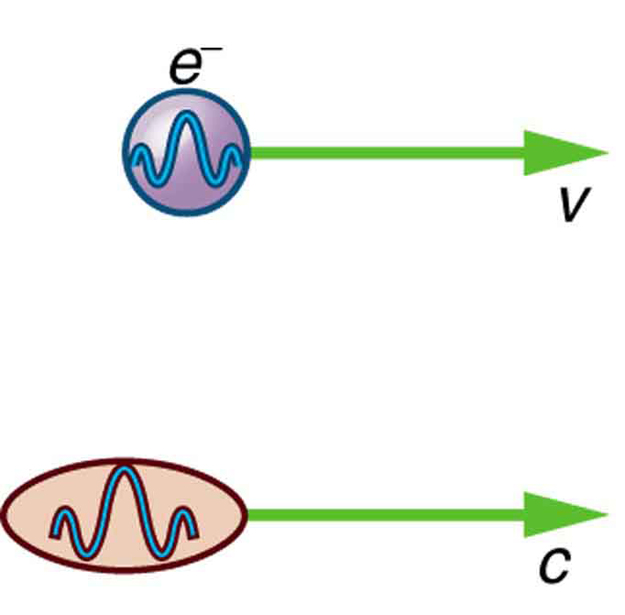There are many submicroscopic particles in nature. Most have mass and are expected to act as particles, or the smallest units of matter. All these masses have wave properties, with wavelengths given by the de Broglie relationship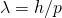. So, too, do combinations of these particles, such as nuclei, atoms, and molecules. As a combination of masses becomes large, particularly if it is large enough to be called macroscopic, its wave nature becomes difficult to observe. This is consistent with our common experience with matter.

Some particles in nature are massless. We have only treated the photon so far, but all massless entities travel at the speed of light, have a wavelength, and exhibit particle and wave behaviors. They have momentum given by a rearrangement of the de Broglie relationship,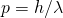. In large combinations of these massless particles (such large combinations are common only for photons or EM waves), there is mostly wave behavior upon detection, and the particle nature becomes difficult to observe. This is also consistent with experience. (See (Figure).)

On a classical scale (macroscopic), particles with mass behave as particles and not as waves. Particles without mass act as waves and not as particles.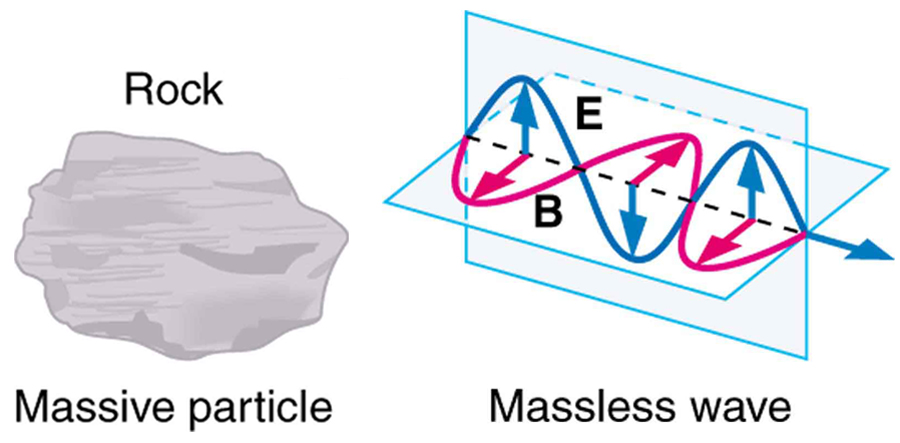The particle-wave duality is a universal attribute. It is another connection between matter and energy. Not only has modern physics been able to describe nature for high speeds and small sizes, it has also discovered new connections and symmetries. There is greater unity and symmetry in nature than was known in the classical era—but they were dreamt of. A beautiful poem written by the English poet William Blake some two centuries ago contains the following four lines:

To see the World in a Grain of Sand

And a Heaven in a Wild Flower

Hold Infinity in the palm of your hand

And Eternity in an hour

### Integrated Concepts

The problem set for this section involves concepts from this chapter and several others. Physics is most interesting when applied to general situations involving more than a narrow set of physical principles. For example, photons have momentum, hence the relevance of Linear Momentum and Collisions. The following topics are involved in some or all of the problems in this section:

Problem-Solving Strategy
1. Identify which physical principles are involved.
2. Solve the problem using strategies outlined in the text.

(Figure) illustrates how these strategies are applied to an integrated-concept problem.

Recoil of a Dust Particle after Absorbing a Photon

The following topics are involved in this integrated concepts worked example:

 Photons (quantum mechanics) Linear Momentum

A 550-nm photon (visible light) is absorbed by a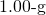particle of dust in outer space. (a) Find the momentum of such a photon. (b) What is the recoil velocity of the particle of dust, assuming it is initially at rest?

Strategy Step 1

To solve an integrated-concept problem, such as those following this example, we must first identify the physical principles involved and identify the chapters in which they are found. Part (a) of this example asks for the momentum of a photon, a topic of the present chapter. Part (b) considers recoil following a collision, a topic of Linear Momentum and Collisions.

Strategy Step 2

The following solutions to each part of the example illustrate how specific problem-solving strategies are applied. These involve identifying knowns and unknowns, checking to see if the answer is reasonable, and so on.

Solution for (a)

The momentum of a photon is related to its wavelength by the equation: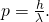Entering the known value for Planck’s constant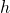and given the wavelength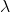, we obtain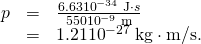Discussion for (a)

This momentum is small, as expected from discussions in the text and the fact that photons of visible light carry small amounts of energy and momentum compared with those carried by macroscopic objects.

Solution for (b)

Conservation of momentum in the absorption of this photon by a grain of dust can be analyzed using the equation: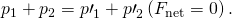The net external force is zero, since the dust is in outer space. Let 1 represent the photon and 2 the dust particle. Before the collision, the dust is at rest (relative to some observer); after the collision, there is no photon (it is absorbed). So conservation of momentum can be written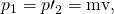where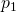is the photon momentum before the collision and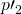is the dust momentum after the collision. The mass and recoil velocity of the dust are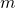and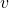, respectively. Solving this for, the requested quantity, yields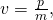where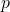is the photon momentum found in part (a). Entering known values (noting that a microgram is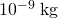) gives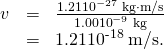Discussion

The recoil velocity of the particle of dust is extremely small. As we have noted, however, there are immense numbers of photons in sunlight and other macroscopic sources. In time, collisions and absorption of many photons could cause a significant recoil of the dust, as observed in comet tails.

### Section Summary

• The particle-wave duality refers to the fact that all particles—those with mass and those without mass—have wave characteristics.
• This is a further connection between mass and energy.

### Conceptual Questions

In what ways are matter and energy related that were not known before the development of relativity and quantum mechanics?

### Problems & Exercises

Integrated Concepts

The 54.0-eV electron in (Figure) has a 0.167-nm wavelength. If such electrons are passed through a double slit and have their first maximum at an angle of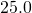, what is the slit separation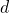?

0.395 nm

Integrated Concepts

An electron microscope produces electrons with a 2.00-pm wavelength. If these are passed through a 1.00-nm single slit, at what angle will the first diffraction minimum be found?

Integrated Concepts

A certain heat lamp emits 200 W of mostly IR radiation averaging 1500 nm in wavelength. (a) What is the average photon energy in joules? (b) How many of these photons are required to increase the temperature of a person’s shoulder by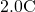, assuming the affected mass is 4.0 kg with a specific heat of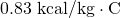. Also assume no other significant heat transfer. (c) How long does this take?

(a)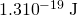(b)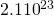(c)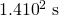Integrated Concepts

On its high power setting, a microwave oven produces 900 W of 2560 MHz microwaves. (a) How many photons per second is this? (b) How many photons are required to increase the temperature of a 0.500-kg mass of pasta by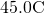, assuming a specific heat of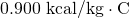? Neglect all other heat transfer. (c) How long must the microwave operator wait for their pasta to be ready?

Integrated Concepts

(a) Calculate the amount of microwave energy in joules needed to raise the temperature of 1.00 kg of soup from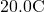to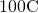. (b) What is the total momentum of all the microwave photons it takes to do this? (c) Calculate the velocity of a 1.00-kg mass with the same momentum. (d) What is the kinetic energy of this mass?

(a)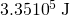(b)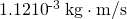(c)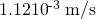(d)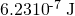Integrated Concepts

(a) What is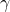for an electron emerging from the Stanford Linear Accelerator with a total energy of 50.0 GeV? (b) Find its momentum. (c) What is the electron’s wavelength?

Integrated Concepts

(a) What isfor a proton having an energy of 1.00 TeV, produced by the Fermilab accelerator? (b) Find its momentum. (c) What is the proton’s wavelength?

(a)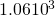(b)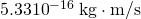(c)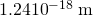Integrated Concepts

An electron microscope passes 1.00-pm-wavelength electrons through a circular aperture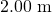in diameter. What is the angle between two just-resolvable point sources for this microscope?

Integrated Concepts

(a) Calculate the velocity of electrons that form the same pattern as 450-nm light when passed through a double slit. (b) Calculate the kinetic energy of each and compare them. (c) Would either be easier to generate than the other? Explain.

(a)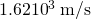(b)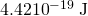for photon,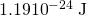for electron, photon energy is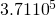times greater

(c) The light is easier to make because 450-nm light is blue light and therefore easy to make. Creating electrons with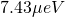of energy would not be difficult, but would require a vacuum.

Integrated Concepts

(a) What is the separation between double slits that produces a second-order minimum at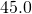for 650-nm light? (b) What slit separation is needed to produce the same pattern for 1.00-keV protons.

(a)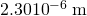(b)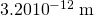Integrated Concepts

A laser with a power output of 2.00 mW at a wavelength of 400 nm is projected onto calcium metal. (a) How many electrons per second are ejected? (b) What power is carried away by the electrons, given that the binding energy is 2.71 eV? (c) Calculate the current of ejected electrons. (d) If the photoelectric material is electrically insulated and acts like a 2.00-pF capacitor, how long will current flow before the capacitor voltage stops it?

Integrated Concepts

One problem with x rays is that they are not sensed. Calculate the temperature increase of a researcher exposed in a few seconds to a nearly fatal accidental dose of x rays under the following conditions. The energy of the x-ray photons is 200 keV, and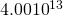of them are absorbed per kilogram of tissue, the specific heat of which is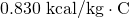. (Note that medical diagnostic x-ray machines cannot produce an intensity this great.)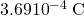Integrated Concepts

A 1.00-fm photon has a wavelength short enough to detect some information about nuclei. (a) What is the photon momentum? (b) What is its energy in joules and MeV? (c) What is the (relativistic) velocity of an electron with the same momentum? (d) Calculate the electron’s kinetic energy.

Integrated Concepts

The momentum of light is exactly reversed when reflected straight back from a mirror, assuming negligible recoil of the mirror. Thus the change in momentum is twice the photon momentum. Suppose light of intensity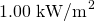reflects from a mirror of area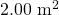. (a) Calculate the energy reflected in 1.00 s. (b) What is the momentum imparted to the mirror? (c) Using the most general form of Newton’s second law, what is the force on the mirror? (d) Does the assumption of no mirror recoil seem reasonable?

(a) 2.00 kJ

(b)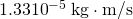(c)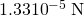(d) yes

Integrated Concepts

Sunlight above the Earth’s atmosphere has an intensity of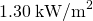. If this is reflected straight back from a mirror that has only a small recoil, the light’s momentum is exactly reversed, giving the mirror twice the incident momentum. (a) Calculate the force per square meter of mirror. (b) Very low mass mirrors can be constructed in the near weightlessness of space, and attached to a spaceship to sail it. Once done, the average mass per square meter of the spaceship is 0.100 kg. Find the acceleration of the spaceship if all other forces are balanced. (c) How fast is it moving 24 hours later?